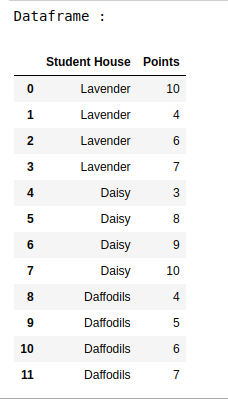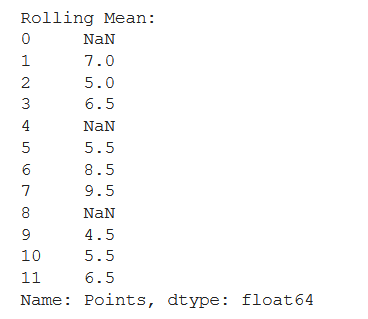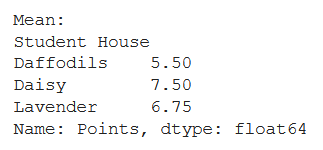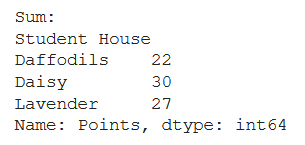Related Articles

# How to apply functions in a Group in a Pandas DataFrame?

• Last Updated : 03 Jan, 2021

In this article, let’s see how to apply functions in a group in a Pandas Dataframe. Steps to be followed for performing this task are –

• Import the necessary libraries.
• Set up the data as a Pandas DataFrame.
• Use apply function to find different statistical measures like Rolling Mean, Average, Sum, Maximum, and Minimum. You can use the lambda function for this.

Below is the implementation-

Let’s create the dataframe.

## Python3

 `#import libraries``import` `pandas as pd`` ` `# set up the data``data_dict ``=` `{``"Student House"``: [``"Lavender"``, ``"Lavender"``, ``"Lavender"``,``                               ``"Lavender"``, ``"Daisy"``, ``"Daisy"``, ``                               ``"Daisy"``, ``"Daisy"``, ``"Daffodils"``, ``                               ``"Daffodils"``, ``"Daffodils"``, ``"Daffodils"``],``              ` `             ``"Points"``: [``10``, ``4``, ``6``, ``7``, ``3``, ``8``, ``9``, ``10``, ``4``, ``5``, ``6``, ``7``]}`` ` `data_df ``=` `pd.DataFrame(data_dict)``print``(``"Dataframe : "``)``data_df`

Output:Example 1:

## Python3

 `# finding rolling mean``rolling_mean ``=` `data_df.groupby(``"Student House"``)[``"Points"``].``apply``(``    ``lambda` `x: x.rolling(center``=``False``, window``=``2``).mean())`` ` `print``(``"Rolling Mean:"``)``print``(rolling_mean)`

Output:Example 2:

## Python3

 `# finding mean``mean ``=` `data_df.groupby(``"Student House"``)[``"Points"``].``apply``(``  ``lambda` `x: x.mean())`` ` `print``(``"Mean:"``)``print``(mean)`

Output:Example 3:

## Python3

 `# finding sum``sum` `=` `data_df.groupby(``"Student House"``)[``"Points"``].``apply``(``  ``lambda` `x: x.``sum``())`` ` `print``(``"Sum:"``)``print``(``sum``)`

Output:Attention geek! Strengthen your foundations with the Python Programming Foundation Course and learn the basics.

To begin with, your interview preparations Enhance your Data Structures concepts with the Python DS Course. And to begin with your Machine Learning Journey, join the Machine Learning – Basic Level Course

My Personal Notes arrow_drop_up